# EM.Ferma Tutorial Lesson 6: Analyzing Permanent Magnets

 Tutorial Project: Analyzing Permanent Magnets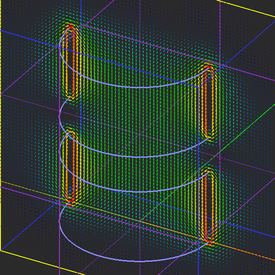Objective: In this project, you will build permanent magnet objects and will analyze their magnetic fields. Concepts/Features: Permanent Magnet Magnetization Vector Magnetic Field Magnetic Vector Potential Minimum Version Required: All versions

## What You Will Learn

In this tutorial you will learn about the third type of magnetic source, i.e. a permanent magnets. You will also learn how to define a new variable and parameterize your physical structure subject to a certain constraint.

## Getting Started

Open the EM.Cube application and switch to EM.Ferma. Start a new project with the following parameters:

 Name EMFerma_Lesson6 Millimeters N/A (keep default) N/A (keep default) N/A (keep default)

## Analyzing a Cylindrical Permanent Magnet

In EM.Ferma, permanent magnets are used as magnetic sources, but they may also be a permeable magnetic material at the same time. Create a permanent magnet source group in the navigation tree. Right-click on the Permanent Magnets item in the navigation tree and select Insert New Permanent Magnet... from the contextual menu. This opens up the New Permanent Magnet dialog. Accept the default name "PM_1" and the default relative permeability of μr = 1. Set the values of the components of the magnetization vector as follows:

Component Definition
Mx 0
My 0
Mz 1000 (A/m)

With the PM_1 group active, draw a cylinder according to the table below:

Part Object Type Material Type Dimensions Coordinates Rotation Angles
Cylinder_1 Cylinder PM_1 radius = 20mm, height = 50mm (0, 0, 0) (0°, 0°, 0°)

Keep the default domain box and the default mesh settings. Define three orthogonal X-, Y- and Z-directed field sensor planes as follows:

Field Sensor Direction Coordinates Plot Type Max. Size Cone Length Ratio Cone Radius Ratio
Sensor_1 X (0, 0, 0) Intensity - - -
Sensor_2 Y (0, 0, 0) Vector 1.5 0.75 0.25
Sensor_3 Z (0, 0, 25mm) Vector 1.5 0.75 0.25

The figures below shows the geometry and mesh of the permanent magnet: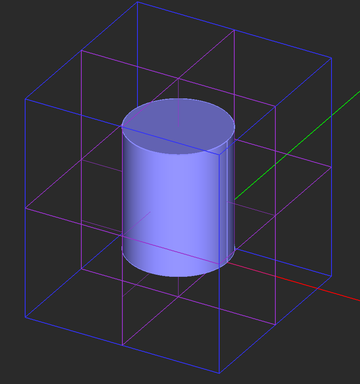The geoemtry of the permanent magnet in the project workspace.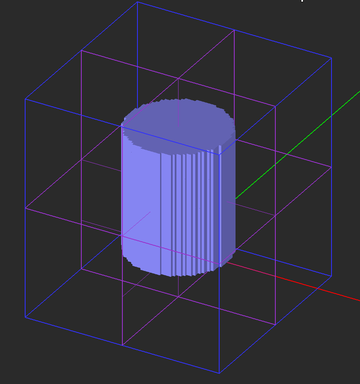The fixed-cell mesh of the permanent magnet.

Run a magnetostatic simulation of your permanent magnet and visualize the field sensor plots. You can see a uniform Z-directed magnetic field inside the magnet.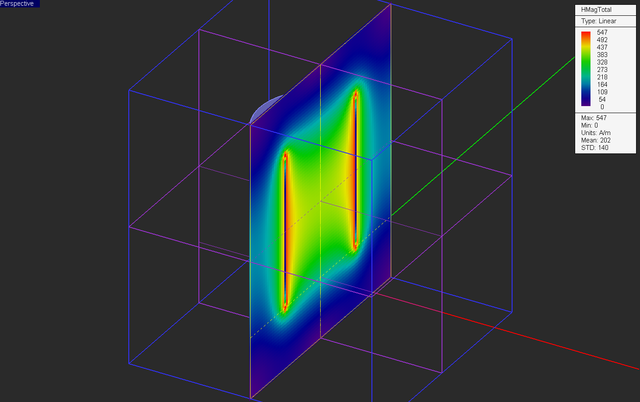The intensity plot of the magnetic field distribution of the permanent magnet in the vertical Sensor_1 plane.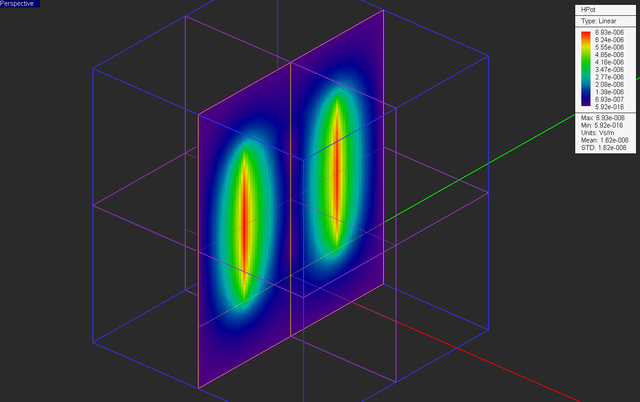The intensity plot of the magnitude of magnetic vector potential distribution of the permanent magnet in the vertical Sensor_1 plane.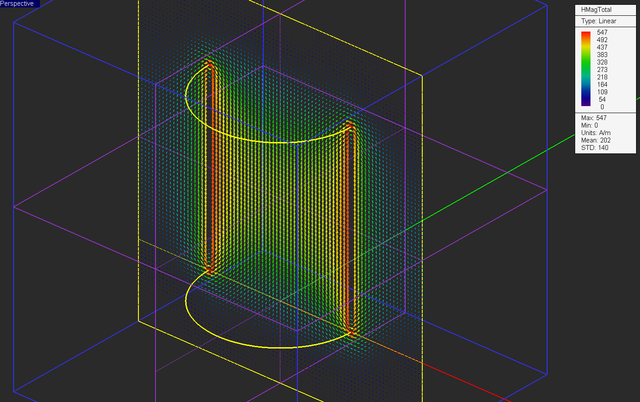The vector plot of the magnetic field distribution of the permanent magnet in the vertical Sensor_2 plane.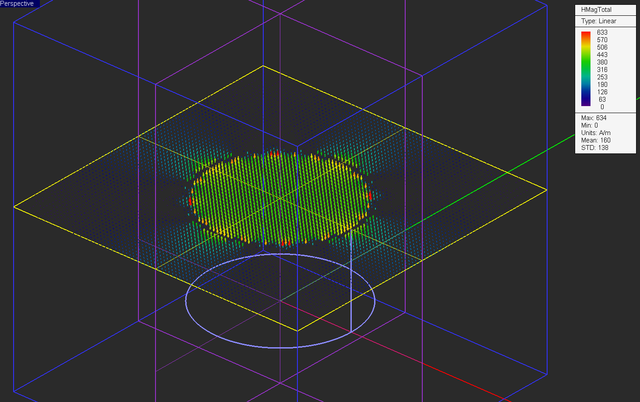The vector plot of the magnetic field distribution of the permanent magnet in the horizontal Sensor_3 plane.

## Simulating the Uniform Magnetic Field Between Two Permanent Magnets

In this part of the tutorial lesson, you will simulate the magnetic field of two parallel cylindrical permanent magnets. With the PM_1 group active, draw two cylinder objects according to the table below. You can simply change the height of the cylinder from the previous part for the first object.

Part Object Type Material Type Dimensions Coordinates Rotation Angles
Cylinder_1 Cylinder PM_1 radius = 20mm, height = 20mm (0, 0, 0) (0°, 0°, 0°)
Cylinder_2 Cylinder PM_1 radius = 20mm, height = 20mm (0, 0, 30mm) (0°, 0°, 0°)

The geometry and mesh of your new physical structure should now look like these: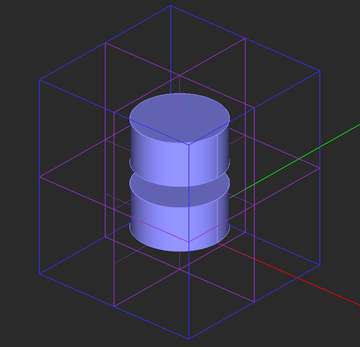The geometry of the two parallel cylindrical magnets.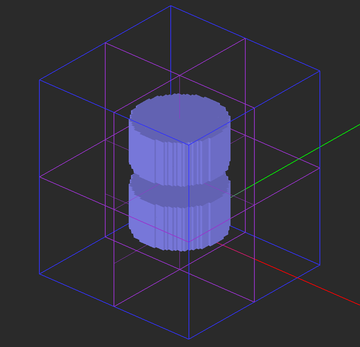The fixed-cell mesh of the two parallel cylindrical magnets.

Run a magnetostatic simulation of your permanent magnets and visualize the field sensor plots. You can see a uniform Z-directed magnetic field in the air region between the two magnets.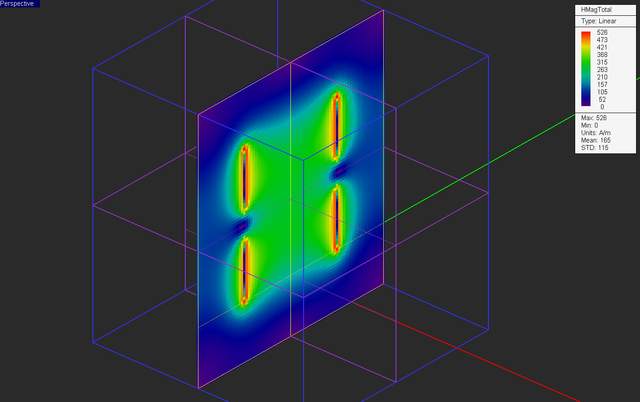The intensity plot of the magnetic field distribution of the two permanent magnets in the vertical Sensor_1 plane.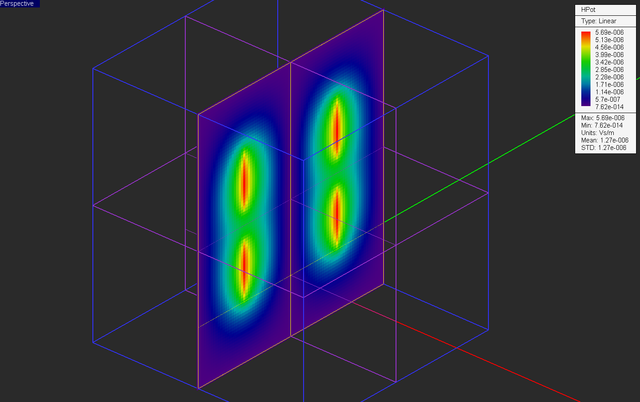The intensity plot of the magnitude of magnetic vector potential distribution of the two permanent magnets in the vertical Sensor_1 plane.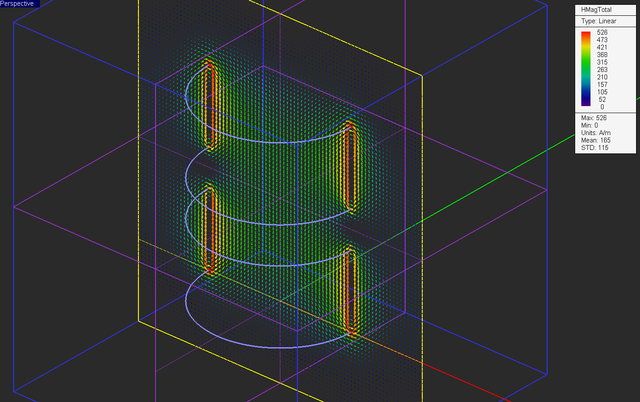The vector plot of the magnetic field distribution of the two permanent magnets in the vertical Sensor_2 plane.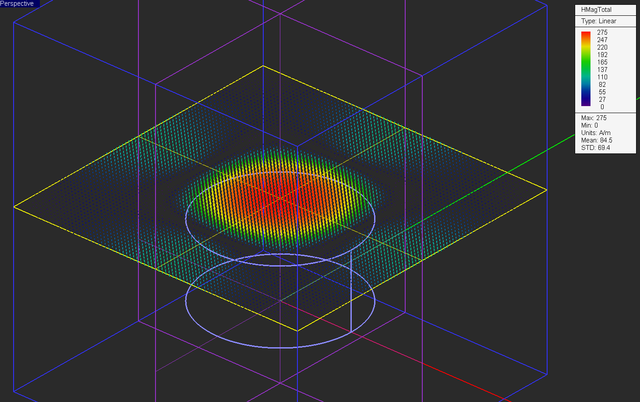The vector plot of the magnetic field distribution of the two permanent magnets in the horizontal Sensor_3 plane.

Also open the data manager and plot the data file "Sensor_1_Z_HTotal.DAT". This plot shows the variation of the magnetic field at the center of the magnets along the Z-axis. At z = 25mm, the magnetic field intensity is 275A/m.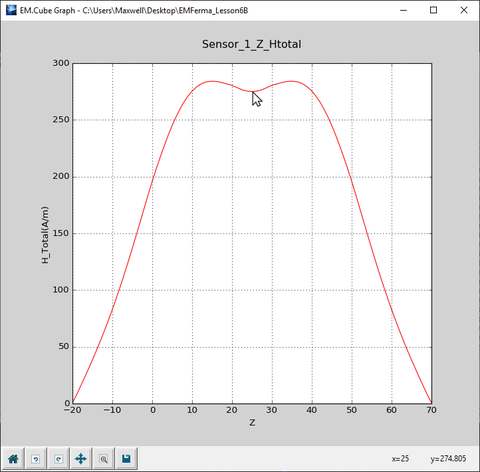The 2D Cartesian graph of variation of the magnetic field of the two magnets along the Z-axis.

## Parameterizing Your Structure Subject to Constraints

In the first part of this tutorial lesson, you simulated a permanent magnet cylinder of height 50mm. In the second part, you simulated two parallel magnet cylinders of height 20mm separated by a spacing of 10mm. The total height of the whole structure is still 50mm. In this part of the tutorial lesson, we are going to treat the spacing between the two cylinders as an air gap and will parameterize its height as a variable called "gap_h". In other words, we will allow the heights of the two cylinders to vary. But we will require the bottom base of "Cyliner_1" to be fixed at Z = 0 and the top base of "Cylinder_2" to be fixed at Z = 50mm.

To define a new variable for your project, open the Variables dialog. The figure below shows that initial default variables list: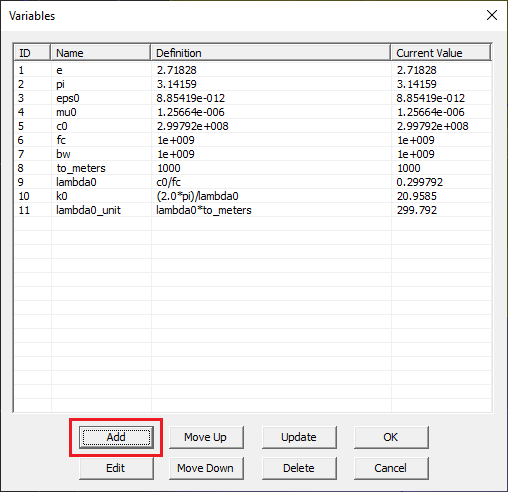The initial variables list showing only the default system variables.

To add a new variable, click the Add button of this dialog. A new "Add Variable" dialog opens up. Enter "gap_h" as the name of the new variable and enter the numeric value "10" as its definition.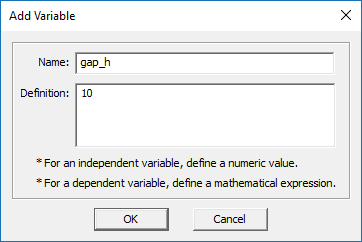Defining a new variable named "gap_h".

Click the OK button and return to the variables dialog. You can see that the name and definition of your new variable have been added to the variables list.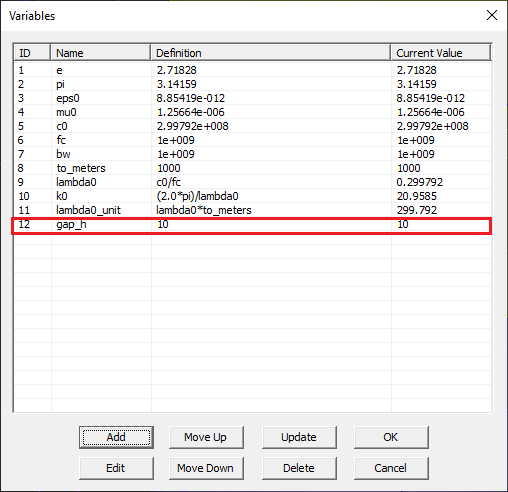The variables list showing the newly added variables "gap_h".

Next, you have to associate this variable with the object properties. This requires a little bit of thinking. The height of the two cylinders must be set equal (50mm - gap_h)/2. This makes the total height of your structure htot = (50mm - gap_h)/2 + gap_h + (50mm - gap_h)/2 = 50mm, as you want. In EM.Cube, the local coordinate system (LCS) of a cylinder is always set up at the center of its bottom base. As you vary "gap_h", the bottom of "Cylinder_1" will always stay fixed at Z = 0, while the bottom of "Cylinder_2" must be located at 50 - (50mm - gap_h)/2 = 25mm + gap_h/2.

Open the property dialog of "Cylinder_1" and set its height equal to "(50-gap_h)/2". Then, open the property dialog of "Cylinder_2", set its height equal to "(50-gap_h)/2" and set its Z-coordinate to "25+gap_h/2".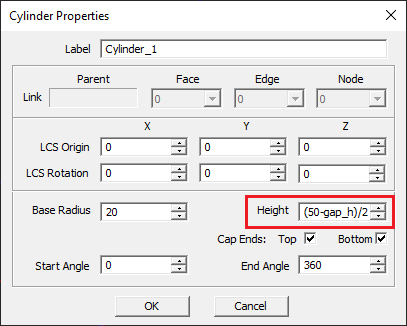Changing the properties of bottom magnet cylinder.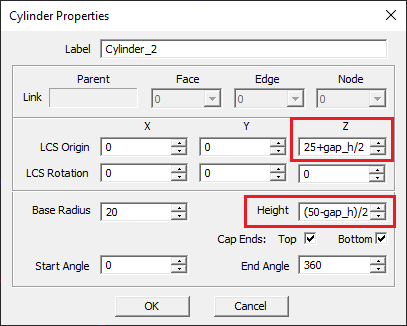Changing the properties of top magnet cylinder.

In Tutorial Lesson 5, you learned how to change the definition or value of an existing variable in the variables dialog. Open the variables dialog again and change the value of "gap_h" to 5mm.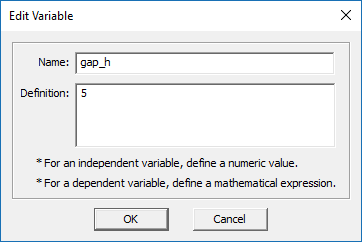Changing the definition of "gap_h" to 5mm.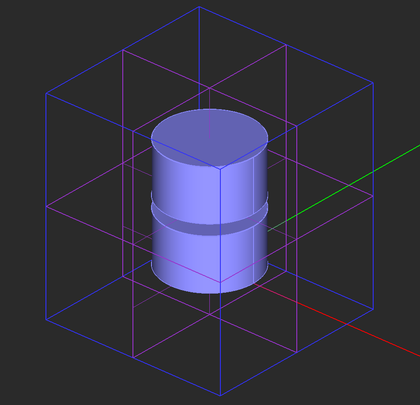The geometry of the two parallel cylindrical magnets with a reduced air gap.

Run a magnetostatic simulation of your new permanent magnets with the reduced air gap and visualize the field sensor plots. Note that the uniform Z-directed magnetic field in the air gap region has increased significantly.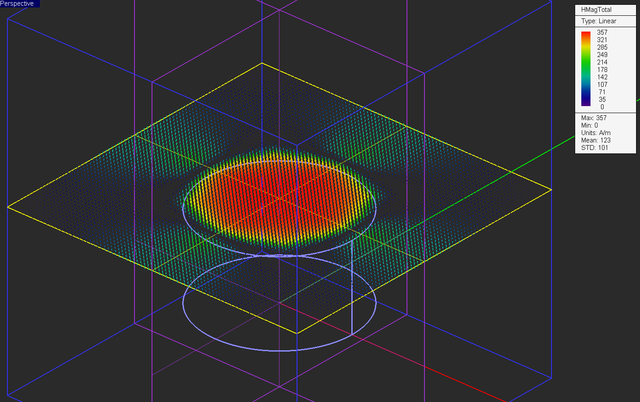The vector plot of the magnetic field distribution of the two permanent magnets with the reduced air gap in the horizontal Sensor_3 plane.

Also open the data manager and plot the data file "Sensor_1_Z_HTotal.DAT". The figure below shows that at z = 25mm, the magnetic field intensity has increased to 357A/m.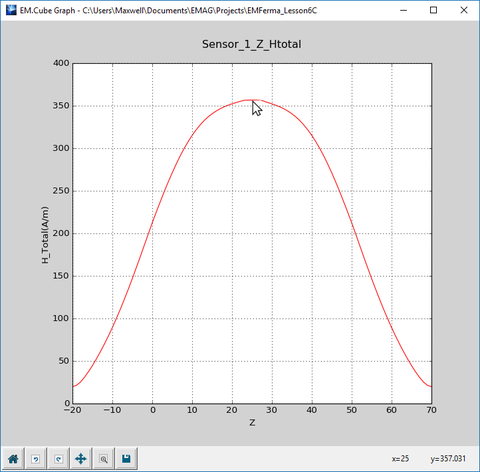The 2D Cartesian graph of variation of the magnetic field of the two magnets with the reduced air gap along the Z-axis.

## Simulating Permeable Magnets

In the previous parts, you assumed that the relative permeability of the permanent magnets was μr = 1. Alnico is a permanent magnet material with μr = 3.6. Open the property dialog of the groups "PM_1" and "PM_2" in the navigation tree and change its relative permittivity to 3.6.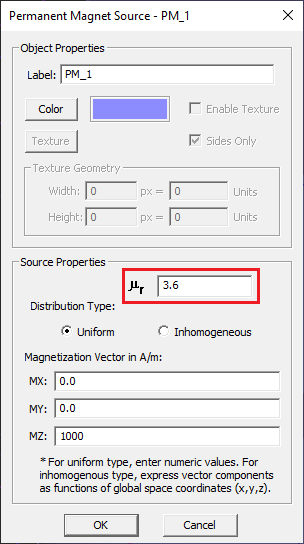Changing the permeability of the permanent magnet.

Keep all the other settings from the previous part and run a magnetostatic simulation of your new Alnico magnets. Visualize the field sensor plots. As you can see from the figures below, the magnetic field intensity inside the permeable material has dropped significantly. Instead, a very strong field has built up in the air gap region as you would have expected.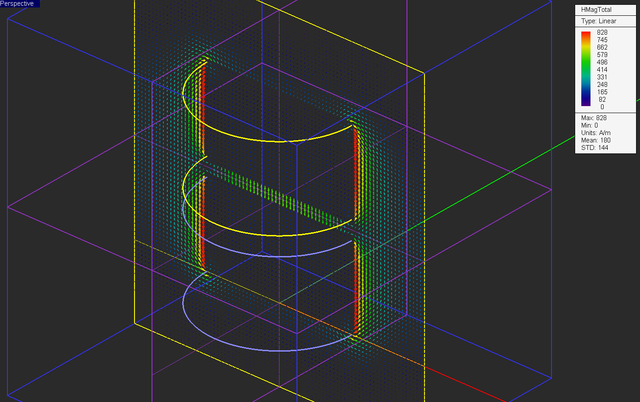The vector plot of the magnetic field distribution of the two Alnico magnets in the vertical Sensor_2 plane.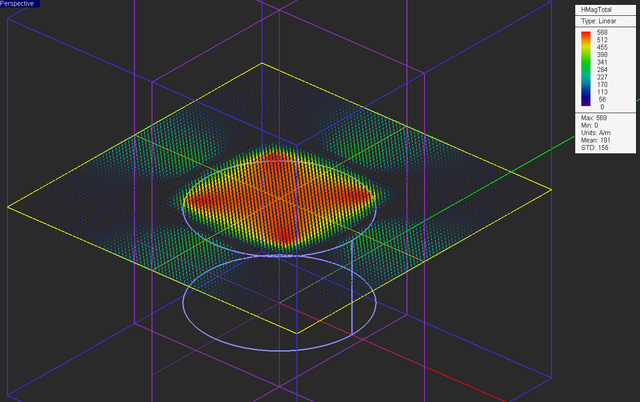The vector plot of the magnetic field distribution of the two Alnico magnets in the horizontal Sensor_3 plane.

Open the data manager and plot the data file "Sensor_1_Z_HTotal.DAT". You can see that along the Z-axis at the center of the two magnets, the field is very much localized and confined to the air gap region and its intensity has increased to a very high value of 634A/m.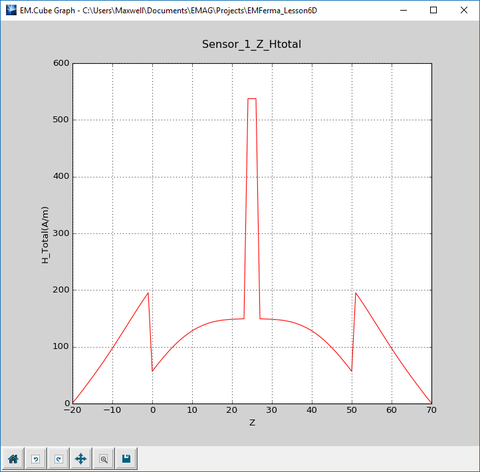The 2D Cartesian graph of variation of the magnetic field of the two magnets with the reduced air gap along the Z-axis.

Also note that the magnetic field undergoes a significant discontinuity at Z = 0, 22.5mm, 27,5mm, and 50mm. These planes are the interfaces between the Alnico media and the air. This is expected as result of the governing boundary conditions between two different magnetic materials. The boundary conditions require that:

$B_{n1} = B_{n2}$

or

$\mu_{r1} H_{n1} = \mu_{r2} H_{n2}$

In the case of your Alnico magnets, this means that

$\frac{H_{z1}}{H_{z2}} = 3.6$

Open and view the contents of the data file "Sensor_1_Z_HTotal.DAT" in the data manager's spreadsheet. You can verify that:

$\frac{|H_z(z=26mm)|}{|H_z(z=27mm)|} = \frac{537.5}{149.4} = 3.60$

$\frac{|H_z(z=51mm)|}{|H_z(z=50mm)|} = \frac{195.1}{56.9} = 3.43$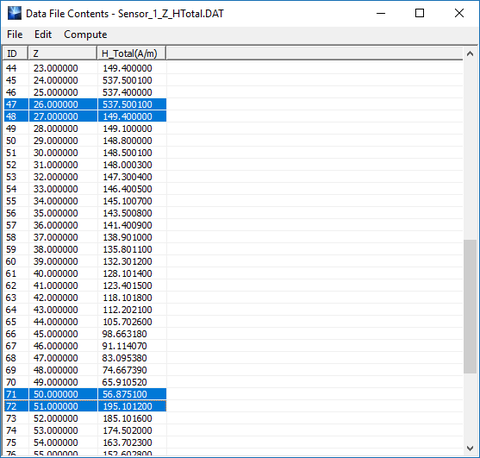The contents of the data file "Sensor_1_Z_HTotal.DAT" shown in data manager's spreadsheet.